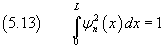## 5. Standing waves.

Standing wave Your browser does not support the HTML5 canvas tag.
input
source
source 1
source 2
amplitude
amplitude 1
amplitude 2
frequency
frequency 1
frequency 2
velocity
velocity 1
velocity 2
ang. di incidenza
ordinal
first harm.
last harm.
larghezza fess.
n. of points
interval
timer
scale

If a point of a medium is fixed, that is if it can't change its physical state, the energy can't overstep it so there can't be flux of energy through it.

To simplify the understanding of this phenomenon, we may consider one-dimensional waves as, for example, those on the strings of musical instruments. The extremities of these strings are fixed to the frame of the instrument, they cannot oscillate like the other points of the string, so their energy is constantly null and there's no flow of energy through them.

Like a particle hitting a hard wall with speed perpendicular to the wall itself, the wave reverses its speed, spreads in the opposite direction, hits the other end by which it is again reflected and so on.

Progressive waves and regressive waves mutually interfere. The result is an interference figure, called standing (or stationary) wave, with nodes at the ends of the string and, possibly, other nodes equidistant from each other. This result implies that the length of the string must exactly contain an integer number of half-wavelengths.

Let L be the length of a string and λ the wavelength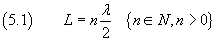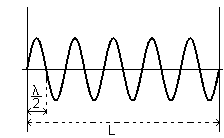You can see some other maybe useful animations in Waves.

If a string (and, in general, any other elastic medium) carries a standing wave, each of its points behaves like an harmonic oscillator. These oscillators have equal angular frequencies ω but different amplitudes, depending on their distance from the extremities. If we let 0 and L be the positions of the extremities, with a convenient choice of the time origin, the physical state of a point P, at position x between 0 and L, is described by the equation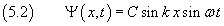in which the amplitude C sin kx must be 0 when x is multiple of the half-wavelength and, in particular, when it is equal to the half-wavelength: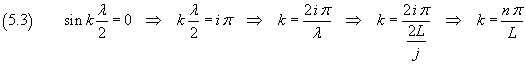Therefore we have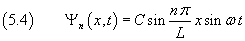The (5.4) is the equation of the n-th standing wave on one-dimensional medium with length L and with nodes at its ends.

The constant C depends on the total energy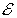owned by the medium.

If we call μ the linear density of a one-dimensional medium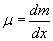from (3.9) the energy of a particle with mass dm swinging with angular frequency ω and amplitude A results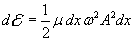In (5.4) the amplitude is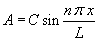, therefore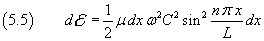The sum of the energies of all the particles of the medium coincides with the total energy owned by the medium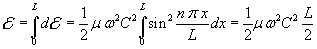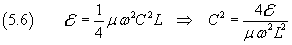The last expression of C2, used in (5.5), gives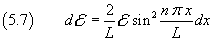and finally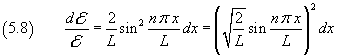(5.8) shows that the relative amount of energy located between x e x+dx is proportional to the square of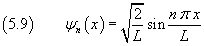that is: the energy density at the position x is given by the square of ψn.

Therefore the square of ψn represents the energy density distribution along the axis x.

(5.9) is independent from μ, so it is a general property of the waves.

So if we let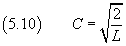and substitute this value in (5.4)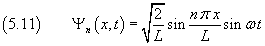we obtain the equation of the n-th stationary wave, which can be carried by a one-dimensional medium with length L, normalized so that the square of its amplitude would represent the energy density.

More simply the equation (5.11) may be written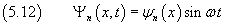with